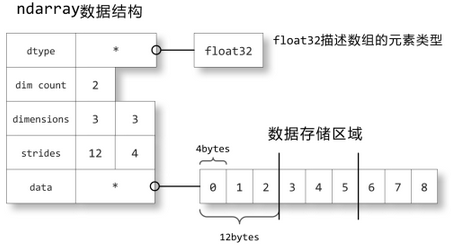ndarray内存结构

from numpy import np

a = np.array([[0,1,2],[3,4,5],[6,7,8]], dtype=np.float32)strides中保存的是当每个轴的下标增加1时，数据存储区中的指针所增加的字节数。例如图中的strides为12,4，即第0轴的下标增加1时，数据的地址增加12个字节：即a[1,0]的地址比a[0,0]的地址要高12个字节，正好是3个单精度浮点数的总字节数；第1轴下标增加1时，数据的地址增加4个字节，正好是单精度浮点数的字节数。

深入dtype

Python内置的基本数据类型，每个类别只有一种，比如只有一种整数int，只有一种浮点数float。对于不需要关心计算机如何存储数据的应用，只提供一种选择是非常方便的。但是对于科学计算而言，这是不够的，为了性能和精度往往需要根据具体场景控制更多的细节。为此，NumPy内置了24种基本类型，基本上可以和C语言的数据类型对应上，其中部分类型对应为Python内置的类型。下表列举了常用NumPy基本类型。

bool_ 兼容Python内置的bool类型
bool8 8位布尔
int_ 兼容Python内置的int类型
int8 8位整数
int16 16位整数
int32 32位整数
int64 64位整数
uint8 无符号8位整数
uint16 无符号16位整数
uint32 无符号32位整数
uint64 无符号64位整数
float_ 兼容Python内置的float类型
float16 16位浮点数
float32 32位浮点数
float64 64位浮点数
str_ 兼容Python内置的str类型

24个scalar types并不是dtype，但是可以作为参数传递给np.dtype()构造函数产生一个dtype对象，如np.dtype(np.int32)。在NumPy中所有需要dtype作为参数的函数都可以使用scalar types代替，会自动转化为对应的dtype类型。

Structured Array

import numpy as np

# list方式：a list of tuples. Each tuple has 2 or 3 elements specifying: 1) The name of the field (‘’ is permitted), 2) the type of the field, and 3) the shape (optional)
persontype = [('name', np.str_), ('age', np.int16), ('weight', np.float32)]

# dict方式：需要指定的键值有names和formats
persontype = np.dtype({
'names': ['name', 'age', 'weight'],
'formats': [np.str_, np.int16, np.float32]
})
a = np.array([("Zhang", 32, 75.5), ("Wang", 24, 65.2)], dtype=persontype)

创建ndarray

# -*- coding: utf-8 -*-
import numpy as np

# 从python的list转换
x = np.array([[1,2.0],[0,0],(1+1j,3.)])

np.zeros((2, 3))
np.arange(2, 3, 0.1) # start, end, step
np.linspace(1., 4., 6) # start, end, num
np.indices((3, 3)) # 返回一个array，元素0是行下标，元素1是列下标；行下标为一个3*3二维array，对应3*3矩阵的行下标；列下标为一个3*3二维array，对应3*3矩阵的列下标

ndtype=[('a',int), ('b', float), ('c', int)]
names = ["A", "B", "C"]
np.genfromtxt("file_name.txt",
delimiter=",",
names=names,
dtype=ndtype,
autostrip=True,
skip_footer=5,
usecols=(0, -1))

# ndarray to list
a = np.array([[1, 2], [3, 4]])
a.tolist()

索引

ndarray索引的基本使用方法示例如下；

x = np.arange(10)
x.shape = (2,5)
x
x[:,0:5:2]

• 虽然x[0,2] = x，但是前者效率比后者高，因为后者在应用第一个索引后需要先创建一个temporary array，然后再应用第二个索引，最后找到目标值。
• 分片操作不会引发copy操作，而是创建原ndarray的view；他们所指向的内存是同一片区域，无论是修改原ndarray还是修改view，都会同时改变二者的值。
• index array和boolean index返回的是copy，不是view。

import numpy as np
a = np.arange(10)
a.shape = (2,5)
print "input a:", a

b = a[0, ]
print "copy b:", b

b = -1
print "modify b:", b
print "final a:", a
## input a: [[0 1 2 3 4]
##  [5 6 7 8 9]]
## copy b: [0 1 2 3 4]
## modify b: [ 0 -1  2  3  4]
## final a: [[ 0 -1  2  3  4]
##  [ 5  6  7  8  9]]

a <- matrix(0:9, nrow=2, byrow=TRUE)
a
##      [,1] [,2] [,3] [,4] [,5]
## [1,]    0    1    2    3    4
## [2,]    5    6    7    8    9
b <- a[1,]
b <- -1
b
##   0 -1  2  3  4
a
##      [,1] [,2] [,3] [,4] [,5]
## [1,]    0    1    2    3    4
## [2,]    5    6    7    8    9

import numpy as np
import time

persontype = [('name', np.str_), ('age', np.int16), ('weight', np.float32)]
a = np.array([("Zhang", 32, 75.5), ("Wang", 24, 65.2)], dtype=persontype)

start = time.clock()
a
end = time.clock()
print "a cost time: %f" % (end-start)

start = time.clock()
a['name']
end = time.clock()
print "a['name'] cost time: %f" % (end-start)

start = time.clock()
a['name']
end = time.clock()
print "a['name'] cost time: %f" % (end-start)

start = time.clock()
a['name']
end = time.clock()
print "a['name'] cost time: %f" % (end-start)
## a cost time: 0.000006
## a['name'] cost time: 0.000020
## a['name'] cost time: 0.000037
## a['name'] cost time: 0.000002